Historical Archive of the Bible Wheel Site

The Bible Wheel had been debunked by its author.
Read all about it: Debunking Myself: What A Long Strange Trip It's Been

Recent Blog ArticlesIn the beginning God created the heaven and the earth.

Genesis 1.1

The first verse of the Holy Bible is the foundation of the Creation Holograph. Its depth knows no limit. Its everlasting perfection glorifies the Lord God beyond measure. The text consists of 7 words and 28 letters: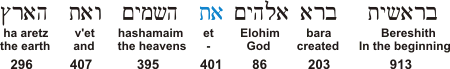At the center of these seven words we see the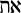(Aleph-Tav). This is the Sign and Seal of the Lord God Almighty, upon which His entire Word is built. The number of letters relates to the number of words by being the seventh Triangular Number:

28 = 1 + 2 + 3 + 4 + 5 + 6 + 7 = T(7)

The sum of the entire verse is the 73rd Triangular Number:

Sum of Genesis 1.1 = 2701 = 37 x 73 = T(73)

The prime Numbers 37 and 73 are geometrically integrated. They form the fourth term in the sequence of Hexagon/Star pairs (the first term being the trivial pair 1 / 1).Note that the numbers 7, 13, 19, 37, and 73 are all primes!. These are the building blocks of the Biblical Holographs, just as the primes are the building blocks of the integers. The interplay of these numbers, their geometric significance, and their integration with Genesis 1.1 is discussed at length in Vernon Jenkin's article called The Lamp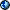.

Hexagon/Star pairs are closely related to Triangular numbers. Their product is always a Triangle, and they can be symmetrically generated from a pair of Triangles, as discussed in Foundation of Creation.

The Number 37 appears in two Hexagon/Star pairs. It is the Star in the 19/37 pair and the Hexagon in the 37/73 pair. This is an extremely rare property. There are only twelve numbers less than seven thousand trillion that can be represented both as a Hexagon and a Star. The Number 37 is the only such number less than a thousand. To find it embedded in the heart of Genesis 1.1 is a clear sign of divine design, especially when coupled with the fact that it is also the geometric basis of the Logos Star which represents God's creative Word in the form of a fractal snowflake. Yet the evidence continues to mount as we note that the substructure of Genesis 1.1 is built upon the Hexagon/Star pair 19/37:

 and the earthV'eth HaAretz = 703 = 19 x 37 = T(37)

This means that Genesis 1.1 exhibits a reiterative structure based on Triangles that result from the product of prime Hexagon/Star pairs - and both of these pairs are associated with the only number less than a thousand that can be represented as both a Hexagon and a Star:

Genesis 1.1 = T(73) =37 x 73 = T(37) + 1998 = 19 x 37 + 1998

Yet there is still more! The number 1998 is three times the 36th Triangle:

Genesis 1.1 = T(73) = T(37) + 3 x T(36)

All these relations - the prime factorization, the Triangular structure, and the Triangular substructure - all result from simple expressions involving elements of the Holographic Generating Set (A = 27, B = 37, C = 73):

 Generating Set Identities for Genesis 1.1 Genesis 1.1 = BC Prime composition of 2701 = T(C) Triangular Structure = T(B)+ 2AB Triangular Substructure

Genesis 1.1 exhibits reiterative structure which is the defining characteristic of Biblical Holographs. Only now the reiteration is both numerical (19/37 and 37/73) and geometric (triangles within triangles and two Hexagon/Star pairs!). This structure has been extensively analyzed by Vernon Jenkins. A good place to start is in his article Arbiters of Truth. The image below summarizes these results: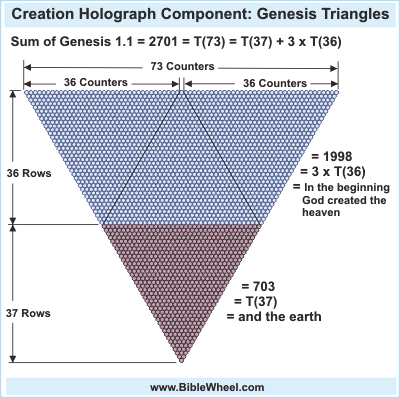Yet this is but the beginning! As discussed at length with regards to the Grace Manifest Holograph, the Number 19 is deeply integrated with the concept of Physical Manifestation. This is displayed with uncanny clarity in the fundamental identity:

 The Earth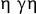hai gai = 19

This integrates with Genesis 1.1:

and the earth (Hebrew) = T(37) = 37 x 19 (The Earth, Greek)

The Numbers 37 and 73 are an extremely significant pair of primes. The essential significance (singlular!) of this palindromic pair of prime numbers is found in these identities generated by the Hebrew word Hokmah (Wisdom):

 WisdomChokmah
37 = 8 + 11 + 13 + 5 (Ordinal Value)73 = 8 + 20 + 40 + 5 (Standard Value)

These identities reveal the supernatural integration of the two fundamental Gematriot:

Vernon Jenkins also notes this pair of identities in his article A Numerical View of Beginnings, where he discusses its relation to the Wisdom presented in association with the numerical analysis of words (Revelation 13.18). Thus, we can understand the sum of Genesis 1.1 as:

Sum of Genesis 1.1 = T(73) = The sum from 1 (Creation) to 73 (Wisdom)

This Wisdom (Hokmah) is associated with creation in Jeremiah 10.12:

He hath made the earth by his power, he hath established the world by his wisdom, and hath stretched out the heavens by his discretion.

Exactly the same pattern - based now on the Hexagon/Star pair 19/37 - is found in the Hebrew word for The Heart:

 The Heart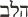HaLev
19 = 5 + 12 + 2 (Ordinal Value)37 = 5 + 30 + 2 (Standard Value)

The same pattern holds for the word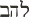(Lahev, Flame) because it is an anagam of "The Heart." Thus, the prime structure of Genesis 1.1 carries strong overtones which are naturally interpreted as The Flaming Heart of Wisdom.

Putting this all together, we arrive at the core of the Creation Holograph, The Heart of Wisdom: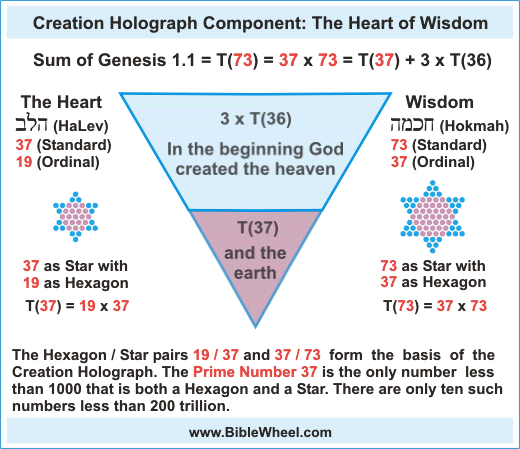This is the foundation of the Creation Holograph. It is, in and of itself, a standing miracle. Yet for all this we have only just begun for John 1.1 extends this structure structure by following a variation of its pattern, so that Genesis 1.1 and John 1.1 are mathematallically, geometrically, and semantically integrated! Endless glory! Such a gift our God has given us!

Next Article: John 1.1.